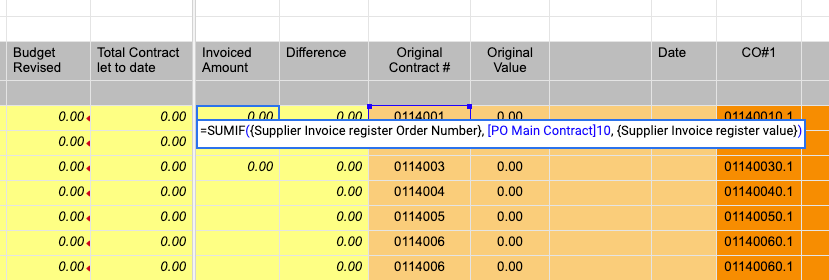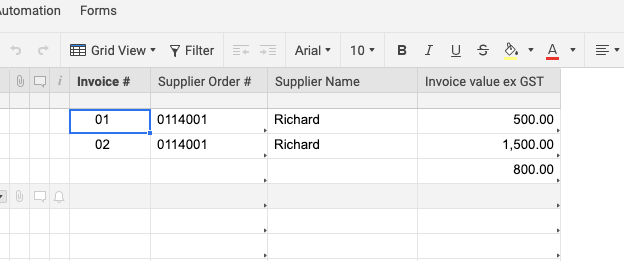# Formulas =SUMIF

Hi,

I need help to solve this puzzle. I'm using this sumif formula

=SUMIF({Supplier Invoice register Order Number}, [PO Main Contract]10, {Supplier Invoice register value})

to sum up the total value of invoices against a particular order number.

I've checked that all columns are formatted as text. See snap shot of actual formula attached.

Cheers

KaneTags:

• What issue exactly are you having?

• For further clarity the formula is returning a zero result instead of 2000

• Hi Paul,

The formula is returning a zero result instead of 2000.

Cheers

Kane

## Help Article Resources

Want to practice working with formulas directly in Smartsheet?

Check out the Formula Handbook template!